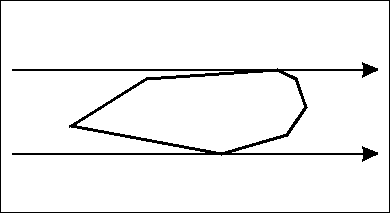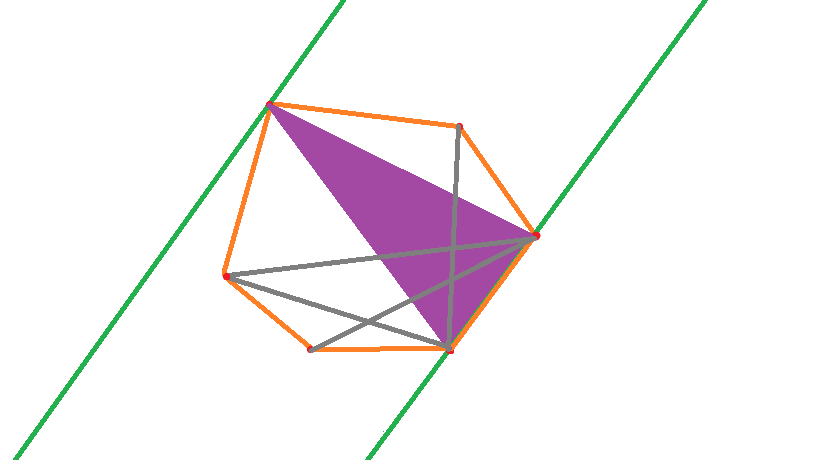## Description:

Bessie, Farmer John's prize cow, has just won first place in a bovine beauty contest, earning the title 'Miss Cow World'. As a result, Bessie will make a tour of N (2 <= N <= 50,000) farms around the world in order to spread goodwill between farmers and their cows. For simplicity, the world will be represented as a two-dimensional plane, where each farm is located at a pair of integer coordinates (x,y), each having a value in the range -10,000 ... 10,000. No two farms share the same pair of coordinates.

Even though Bessie travels directly in a straight line between pairs of farms, the distance between some farms can be quite large, so she wants to bring a suitcase full of hay with her so she has enough food to eat on each leg of her journey. Since Bessie refills her suitcase at every farm she visits, she wants to determine the maximum possible distance she might need to travel so she knows the size of suitcase she must bring.Help Bessie by computing the maximum distance among all pairs of farms.

## Input:

* Line 1: A single integer, N

* Lines 2..N+1: Two space-separated integers x and y specifying coordinate of each farm

## Output:

* Line 1: A single integer that is the squared distance between the pair of farms that are farthest apart from each other.

4
0 0
0 1
1 1
1 0

## Sample Output:

2

### 题目链接## AC代码:

// submit C++
#include <cstdio>
#include <iostream>
#include <cmath>
#include <vector>
#include <algorithm>
using namespace std;

typedef double db;
const int maxn = 5e4 + 5;
const db eps = 1e-9;

int Sgn(db Key) {return fabs(Key) < eps ? 0 : (Key < 0) ? -1 : 1;}
int Cmp(db Key1, db Key2) {return Sgn(Key1 - Key2);}
struct Point {
db X, Y;
Point() {}
Point(db _X, db _Y) {
X = _X;
Y = _Y;
}
};
typedef Point Vector;
Vector operator - (Vector Key1, Vector Key2) {return Vector(Key1.X - Key2.X, Key1.Y - Key2.Y);}
Vector operator + (Vector Key1, Vector Key2) {return Vector(Key1.X + Key2.X, Key1.Y + Key2.Y);}
db operator * (Vector Key1, Vector Key2) {return Key1.X * Key2.X + Key1.Y * Key2.Y;}
db operator ^ (Vector Key1, Vector Key2) {return Key1.X * Key2.Y - Key1.Y * Key2.X;}
db DisPointToPoint(Point Key1, Point Key2) {return sqrt((Key1 - Key2) * (Key1 - Key2));}
db DisPointToPoint2(Point Key1, Point Key2) {return (Key1 - Key2) * (Key1 - Key2);}
typedef vector<Point> Polygon;

int N;
Point points[maxn];
Polygon ConvexHull;
db Ans;

void RotateCaliper() {
Ans = -1e20;
if (ConvexHull.size() == 3) {
if (Cmp(DisPointToPoint2(ConvexHull, ConvexHull), Ans) > 0) Ans = DisPointToPoint2(ConvexHull, ConvexHull);
if (Cmp(DisPointToPoint2(ConvexHull, ConvexHull), Ans) > 0) Ans = DisPointToPoint2(ConvexHull, ConvexHull);
if (Cmp(DisPointToPoint2(ConvexHull, ConvexHull), Ans) > 0) Ans = DisPointToPoint2(ConvexHull, ConvexHull);
return;
}
int Cur = 2, Size = ConvexHull.size();
for (int i = 0; i < Size; ++i) {
while (Cmp(fabs((ConvexHull[i] - ConvexHull[(i + 1) % Size]) ^ (ConvexHull[Cur] - ConvexHull[(i + 1) % Size])), fabs((ConvexHull[i] - ConvexHull[(i + 1) % Size]) ^ (ConvexHull[(Cur + 1) % Size] - ConvexHull[(i + 1) % Size]))) < 0) Cur = (Cur + 1) % Size;
if (Cmp(DisPointToPoint2(ConvexHull[i], ConvexHull[Cur]), Ans) > 0) Ans = DisPointToPoint2(ConvexHull[i], ConvexHull[Cur]);
}
}

bool SortRule(const Point &Key1, const Point Key2) {
db Temp = (Key1 - points) ^ (Key2 - points);
if (Sgn(Temp) > 0) return true;
else if (Sgn(Temp) == 0 && Cmp(DisPointToPoint(Key2, points), DisPointToPoint(Key1, points)) > 0) return true;
return false;
}

Polygon GrahamScan() {
Polygon Ans;
if (N < 3) {
for (int i = 0; i < N; ++i) Ans.push_back(points[i]);
return Ans;
}
int Low = 0;
for (int i = 0; i < N; ++i)
if (points[i].Y < points[Low].Y || (points[i].Y == points[Low].Y && points[i].X < points[Low].X))
Low = i;
swap(points, points[Low]);
sort(points + 1, points + N, SortRule);
Ans.push_back(points);
for (int i = 1; i < N; ++i) {
while (Ans.size() >= 2 && Sgn((Ans.back() - Ans[Ans.size() - 2]) ^ (points[i] - Ans[Ans.size() - 2])) <= 0)
Ans.pop_back();
Ans.push_back(points[i]);
}
return Ans;
}

int main(int argc, char *argv[]) {
scanf("%d", &N);
for (int i = 0; i < N; ++i) scanf("%lf%lf", &points[i].X, &points[i].Y);
ConvexHull = GrahamScan();
if (ConvexHull.size() == 2) {
printf("%.0lf\n", DisPointToPoint2(ConvexHull, ConvexHull));
return 0;
}
RotateCaliper();
printf("%.0lf\n", Ans);
return 0;
}
Last modification：March 27th, 2019 at 11:15 pm